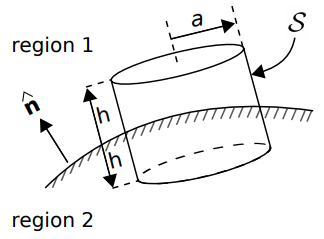# Boundary Conditions on the Magnetic Flux Density (B)

In homogeneous media, electromagnetic quantities vary smoothly and continuously. At an interface between dissimilar media, however, it is possible for electromagnetic quantities to be discontinuous. Continuities and discontinuities in fields can be described mathematically by boundary conditions and used to constrain solutions for fields away from these interfaces.

In this section, we derive the boundary condition on the magnetic flux density

at a smooth interface between two material regions, as shown in Figure 7.10.1.1 The desired boundary condition may be obtained from Gauss’ Law for Magnetic Fields (GLM; Section 7.2):

where

is any closed surface. Let

take the form of cylinder centered at a point on the interface, and for which the flat ends are parallel to the surface and perpendicular to

, as shown in Figure 7.10.1. Let the radius of this cylinder be

, and let the length of the cylinder be

.Figure 7.10.1: Determination of the boundary condition on B at the interface between material regions © CC BY SA 4.0.

From GLM, we have

Now let us reduce

and

together while (1) maintaining a constant ratio

and (2) keeping

centered on the interface. Because

, the area of the side can be made negligible relative to the area of the top and bottom. Then, as

, we are left with

As the area of the top and bottom sides become infinitesimal, the variation in

over these areas becomes negligible. Now we have simply:

where

and

are the magnetic flux densities at the interface but in regions 1 and 2, respectively, and

is the area of the top and bottom sides. Note that the orientation of

is important – we have assumed

points into region 1, and we must now stick with this choice. Thus, we obtain

where, as noted above,

points into region 1.

### Summarizing

The normal (perpendicular) component of

across the boundary between two material regions is continuous.

It is worth noting what this means for the magnetic field intensity

. Since

, it must be that

The normal (perpendicular) component of

across the boundary between two material regions is discontinuous if the permeabilities are unequal.

## Footnotes

• 1

It may be helpful to note the similarity (duality, in fact) between this derivation and the derivation of the associated boundary condition on D presented in Section 5.18.

Ellingson, Steven W. (2018) Electromagnetics, Vol. 1. Blacksburg, VA: VT Publishing. https://doi.org/10.21061/electromagnetics-vol-1 CC BY-SA 4.0Swipe left and right to change pages.